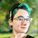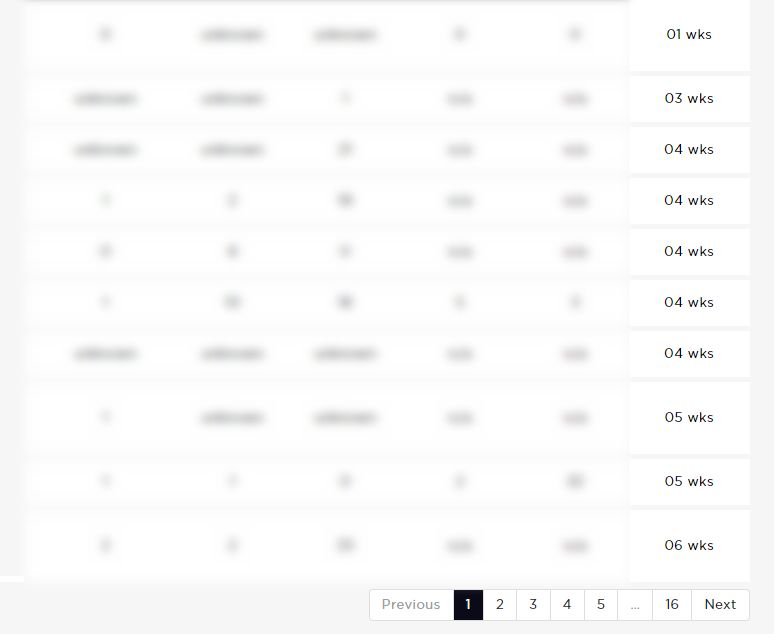## CMS DevelopmentContributeur de premier rang

## Displaying 'length' of answers from a calculation

SOLVE

I've created a table that pulls in HubDB data between 2 date columns;

{% set exchanged = unixtimestamp(row.exchange_date) %}

{% set reservation = unixtimestamp(row.reservation_date) %}

{% set exchanged_date = (exchanged - reservation)//24//60//60//1000 %}

Above calculates the number of days between the 2 column entries.

{% set total_weeks = (exchanged_date / 7 ) | round %}

Then I use the number of days and round off to show how many weeks...

{% for row in table %}

{% endfor %}

The above works, but now I want to display a bar graph that shows how many show 4 weeks, 5 weeks, 6 weeks etc. How do I calculate this? I tried the below but it didn't work:

{% set test1 = (total_weeks, "equalto", "4") | length %}

Any ideas would be appreciated

1 Accepted solutionSolution## Displaying 'length' of answers from a calculation

SOLVE

@ben-duchy You would want to push the calculated weeks into an array and then use selectattr.

``````{% set table = hubdb_table_rows(3958688) %}

{% set array = [] %} // create array variable

{% for row in table %}
{% set exchanged = unixtimestamp(row.exchange_date) %}
{% set reservation = unixtimestamp(row.reservation_date) %}
{% set exchanged_date = (exchanged - reservation)//24//60//60//1000 %}

{% set total_weeks = (exchanged_date / 7 ) | round %}

{% do array.append({"weeks": total_weeks}) %} // append number of weeks to array
{% endfor %}

{% set wks1 = array|selectattr("weeks","equalto","1")|length %} // find length with selectattr
{{ wks1 }}``````

And if you don't wanna write a whole bunch of sets for every possible number of weeks you could write a for loop with a range

``````{% for x in range(0,6) %}
{% set wks = array|selectattr("weeks","equalto",x)|length %}
{{ wks }}
{% endfor %}``````

``````{% set wks_total = {"total": "0"} %}

{% for x in range(-6,0) %}
{% set wks = array|selectattr("weeks","equalto",x)|length %}

{% set new_total = wks_total.total|int + wks|int %}
{% do wks_total.update({"total": new_total}) %}
{% endfor %}

{{ wks_total.total }}``````### Alyssa Wilie

#### Web Developer at LyntonWebContributeur de premier rang

## Displaying 'length' of answers from a calculation

SOLVE

Hi @amwilie, you're an absolute diamond! Thank you so much 😁Contributeur de premier rang

## Displaying 'length' of answers from a calculation

SOLVE

Hi @amwilie,

Good spot! You're correct that the loop does need to cover everything as you stated. This works if you want to display all the information inside a table (see below), but what I now need to do is calculate how may rows show 01 wks or 02 wks etc, another words...

• 01 wks = 1
• 03 wks = 1
• 04 wks = 5
• 05 wks = 2If I was pulling data directly from HubDB, I would use the selectattr method for example:

{% set table = hubdb_table_rows(xxxxxxx, queryparam) %}
{% set wks1 = table | selectattr ("weeks.name","equalto","one") | length %}

{% set wks2 = table | selectattr ("weeks.name","equalto","two") | length %}

{% set wks_total = (wks1 + wks2) %}

The 2 numbers then get added together within my page using {{wks_total}}

Problem is that unlike my example above, the 'wks' column that I want to use doesn't exist in HubDB. The 'wks' column in the table above is a calculation between 2 HubDB columns, therefore how do I split a hubl calculation into more filtered data?Solution## Displaying 'length' of answers from a calculation

SOLVE

@ben-duchy You would want to push the calculated weeks into an array and then use selectattr.

``````{% set table = hubdb_table_rows(3958688) %}

{% set array = [] %} // create array variable

{% for row in table %}
{% set exchanged = unixtimestamp(row.exchange_date) %}
{% set reservation = unixtimestamp(row.reservation_date) %}
{% set exchanged_date = (exchanged - reservation)//24//60//60//1000 %}

{% set total_weeks = (exchanged_date / 7 ) | round %}

{% do array.append({"weeks": total_weeks}) %} // append number of weeks to array
{% endfor %}

{% set wks1 = array|selectattr("weeks","equalto","1")|length %} // find length with selectattr
{{ wks1 }}``````

And if you don't wanna write a whole bunch of sets for every possible number of weeks you could write a for loop with a range

``````{% for x in range(0,6) %}
{% set wks = array|selectattr("weeks","equalto",x)|length %}
{{ wks }}
{% endfor %}``````

``````{% set wks_total = {"total": "0"} %}

{% for x in range(-6,0) %}
{% set wks = array|selectattr("weeks","equalto",x)|length %}

{% set new_total = wks_total.total|int + wks|int %}
{% do wks_total.update({"total": new_total}) %}
{% endfor %}

{{ wks_total.total }}``````### Alyssa Wilie

#### Web Developer at LyntonWeb## Displaying 'length' of answers from a calculation

SOLVE

Hey @ben-duchy

Don't know if this is your whole code so correct me if I'm wrong - but do you have the calculations inside the same for loop as where you're using {{ total_weeks }}? Because if not it's not going to be able to associate the {{ total_weeks }} with the calculations. This worked for me :

``````{% set table = hubdb_table_rows(XXXXXX) %}

{% for row in table %}
{% set exchanged = unixtimestamp(row.exchange_date) %}
{% set reservation = unixtimestamp(row.reservation_date) %}
{% set exchanged_date = (reservation - exchanged)//24//60//60//1000 %}
{% set total_weeks = (exchanged_date / 7 ) | round %}